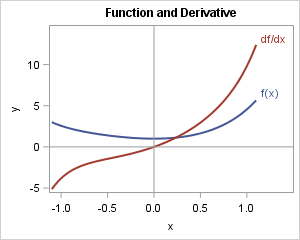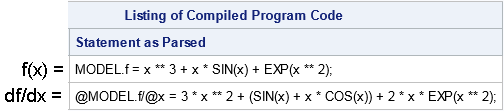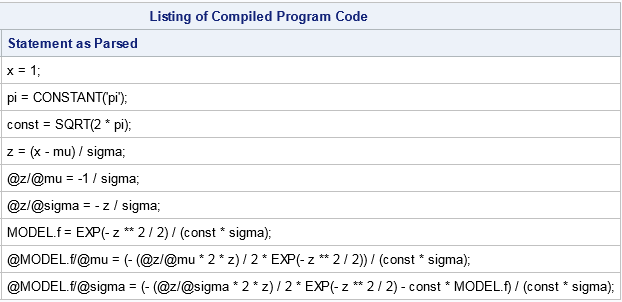Did you know that you can get SAS to compute symbolic (analytical) derivatives of simple functions, including applying the product rule, quotient rule, and chain rule? SAS can form the symbolic derivatives of single-variable functions and partial derivatives of multivariable functions. Furthermore, the derivatives are output in a form that can be pasted into a SAS program. The trick is to use PROC NLIN with the LIST option.

In SAS/IML, the nonlinear optimization routines will use analytical derivatives if they are provided; otherwise, they will automatically generate numerical finite-difference approximations to the derivatives. I rarely specify derivatives because it can be time-consuming and prone to error. But recently I realized that PROC NLIN and other SAS procedures automatically generate symbolic derivatives, and you can "trick" the procedure into displaying the derivatives so that they can be used in other applications. To get PROC NLIN to output symbolic derivatives, do the following:

• Create a data set to use for the DATA= option of PROC NLIN.
• List the variables that you want to take derivatives of on the PARMS statement. In open code, assign a value to constants that appear in the function.
• Specify the function to differentiate on the MODEL statement.

### Symbolic derivatives of functions of one variableHere is a one-variable example. Suppose you want to take the derivative of
f(x) = x3 + x sin(x) + exp( x2 ).
The function and its derivative is shown to the right.

The following SAS statements create a "fake" data set that defines a variable named F. The call to PROC NLIN sets up the problem. The LIST option on the PROC NLIN statement generates the 'ProgList' table, which contains the derivative function:

```data _dummy; f = 1; /* name of function */ run;   proc nlin data=_dummy list; parms x=0; /* list variables */ model f = x**3 + x*sin(x) + exp(x**2); /* define function */ ods select ProgList; /* output symbolic derivatives */ run;```The output shows the expressions for the function ("MODEL.f") and the derivative with respect to x. The derivative df/dx is written as "@MODEL.f/@x", where the symbol '@' is used for the 'd'. You can see that SAS took the simple derivative of the x3 term, applied the product rule to the x*sin(x) term, and applied the chain rule to the exp( x2 ) term. You can also see that the expressions are written in SAS notation, with "**" indicating the power operator. SAS functions are written in upper case (SIN, COS, and EXP).

### Symbolic partial derivatives of multivariate functions

In exactly the same way, you can obtain partial derivatives. In a partial derivative, all variables are held constant except one. Suppose you have the function for the normal density,
f(x) = 1/(sqrt(2 π) σ) exp( -(x - μ)**2 / (2 σ**2) )
Suppose that you want to compute the partial derivatives ∂f/∂μ and ∂f/∂σ. To compute these derivatives, list μ and σ on the PARMS statement and assign values to the other symbols (x and π) that appear in the function. It does not matter what value you assign to the x and π, you are just preventing PROC NLIN from complaining that there are unassigned variables. You could assign the value 1 to both variables, but I prefer to tell the procedure that π equals 3.14. In the following program, note that PROC NLIN reuses the fake data set that defines the F variable:

```proc nlin data=_dummy list; parms mu=0 sigma=1; /* list variables */ x=1; pi = constant('pi'); /* assign arbitrary values to constants */ model f = exp( -(x-mu)**2 / (2*sigma**2) ) / (sqrt(2*pi)*sigma); /* define function */ ods select ProgList; /* output symbolic derivatives */ run;```The derivative with respect to σ requires using the chain rule and the quotient rule. Notice that the derivative includes a call to the original function ("MODEL.f"). Notice also that there is repetition of various expressions such as -(x - μ)**2 / (2 σ**2). If you copy and paste these expressions into a SAS program that computes the derivative, the computation will be slightly inefficient because the program will evaluate the same expression multiple times. One way to improve the efficiency is to collect sub-expressions into a temporary variable, as shown in the next section.

### Sub-expressions and the chain rule

An experienced statistician will recognize that the expression z = (x - μ) / σ arises naturally as the standardized variable. Using such an expression might simplify the derivatives.

The following program computes the same derivatives as before, except this time the programmer defines the expression z = (x - μ) / σ and uses z in the function. When SAS takes the derivative, it computes dz/dμ and dz/dσ as part of the computation, as shown below:

```proc nlin data=_dummy list; parms mu=0 sigma=1; /* list variables */ x=1; pi = constant('pi'); /* assign arbitrary values to constants */ const = sqrt(2*pi); z = (x-mu) / sigma; /* intermediate expression */ model f = exp(-z**2 / 2) / (const*sigma); /* define function */ ods select ProgList; /* output symbolic derivatives */ run;```Notice that the derivatives use several sub-expressions such as dz/dμ and dz/dσ. This computation is more efficient than the previous because it reuses previously computed quantities. For another example, define f1 = 1 / sqrt(2*pi*sigma) and f2 = exp(-z**2 / 2) and define the MODEL as f = f1*f2. You will see that each term in the derivative is efficiently evaluated in terms of previously expressions.

### Discussion of symbolic derivatives

As I said previously, I do not usually specify analytical derivatives for my optimizations. I find that numerical derivatives (which are automatically computed) are fast and accurate for 99% of the optimizations that I perform.

Furthermore, some of the objective functions that I optimize do not have analytical derivatives. Others are matrix computations for which it would be difficult and time-consuming to write down the analytical derivative. (For an example, see the article on the derivative of the bivariate normal cumulative distribution.)

If you decide to specify an analytical derivative in SAS, make sure that the derivative is correct and efficient. The technique in this article will help to ensure that the derivative is correct. By strategically defining sub-expressions as in the previous section, you can help SAS generate derivatives that are computationally efficient.

Be aware that the derivatives are generated by applying the chain rule, product rule, and quotient rule without any algebraic simplifications of the result. Thus the result can be unnecessarily complicated. For an example, ask SAS to display the derivative of log( (1+x)/(1-x) ) / 2. The naive result is complicated. If you rewrite the function as log(1+x)/2 - log(1-x)/2, then the derivative is much simpler. However, in neither case will SAS simplify the derivative to obtain 1 / (1-x**2).

For more of my thoughts on using derivatives in SAS, see "Two hints for specifying derivatives." What about your thoughts? What do you think about using PROC NLIN to generate automatic derivatives of simple functions? Do you think this technique will be useful to you?

The post Symbolic derivatives in SAS appeared first on The DO Loop.